Name:    2nd Grade Math Proficient Test 9

Multiple Choice
Identify the choice that best completes the statement or answers the question.

1.

Round to the nearest hundred.
862
 a. 800 d. 862 b. 860 e. 960 c. 900

2.

Roman Numerals I, V, and X have the value of _________.
 a. 10, 15, 20 d. 1, 5, 10 b. 5, 10, 15 e. 1, 25, 50 c. 1, 10, 20

3.

Which number can be divided by 5 with no remainder?
 a. 7,319 d. 1,042 b. 2,980 e. 6,543 c. 5,287

4.

What is the greatest common factor of 54 and 72?
 a. 9 d. 1 b. 2 e. 12 c. 3

5.

What are the two common multiples of 4 and 6?
 a. 18, 20 d. 16, 28 b. 30, 40 e. 12, 24 c. 8, 10

6.

Number Sense and Numeration – Decimals – RIT 191 – 200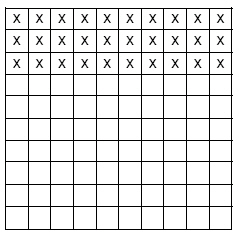What decimal does the shaded area represent?
 a. 0.33 d. .3 b. 0.03 e. 3.3 c. 1.3

7.

What is the value of the digit 4 in the number .246?
 a. 1/4 d. 4/100 b. 4/1 e. 100/4 c. 4/1000

8.

Number Sense and Numeration – Exponents – RIT 191 – 200

How is (5 x 5 x 5 x 5) represented in exponential form?
 a.d.b.e.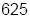c.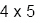9.

Number Sense and Numeration – Fractions – RIT 191 – 200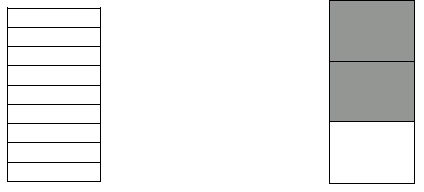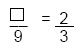a. 7 d. 6 b. 4 e. 3 c. 8

10.

2/4 = __/28
 a. 12 d. 14 b. 6 e. 32 c. 7

11.

Change 38/6 to a mixed number in simplest form.
 a. 6 2/6 d. 3 6/8 b. 6/38 e. 6 c. 6 1/3

12.

What is the LCD (least common denominator) of 4 and 3?
 a. 12 d. 8 b. 7 e. 18 c. 11

13.

1/5 expressed as a decimal =
 a. 0.25 d. .05 b. 0.2 e. .15 c. 0.5

14.

Which fractions are equal ratios? (Use the cross product method)
 a. 5/6, 9/12 d. 5/10, 7/14 b. 7/21, 6/9 e. 5/7, 10/15 c. 4/3, 9/16

15.

Number Sense and Numeration – Ordering, Equalities, Inequalities –
RIT 191 – 200

Which expression is correct?
 a. 799,265 < 786,362 d. 698,125 < 672,986 b. 851,462 > 815,467 e. 375,682 > 378,986 c. 989,468 > 998,468